4626.0.55.001 - Environmental views and behaviour, 2011-12
Latest ISSUE Released at 11:30 AM (CANBERRA TIME) 11/12/2012
Page tools: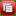Print AllRSSSearch this Product

TECHNICAL NOTE DATA QUALITY

RELIABILITY OF THE ESTIMATE

1 Since the estimates in this publication are based on information obtained from a sample, they are subject to sampling variability. That is, they may differ from those estimates that would have been produced if all dwellings had been included in the survey. One measure of the likely difference is given by the standard error (SE), which indicates the extent to which an estimate might have varied by chance because only a sample of dwellings (or households) was included. There are about two chances in three (67%) that a sample estimate will differ by less than one SE from the number that would have been obtained if all dwellings had been included, and about 19 chances in 20 (95%) that the difference will be less than two SEs.

2 Another measure of the likely difference is the relative standard error (RSE), which is obtained by expressing the SE as a percentage of the estimate: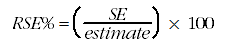3 RSEs for estimates from 2011-12 Environmental Views and Behaviour have been calculated using the Jackknife method of variance estimation. This involves the calculation of 30 'replicate' estimates based on 30 different subsamples of the obtained sample. The variability of estimates obtained from these subsamples is used to estimate the sample variability surrounding the estimate.

4 A Data Cube (spreadsheet) containing all tables produced for this publication and the calculated RSEs for each of the estimates is available from the Downloads tab of the publication. For illustrative purposes the RSEs for Table 6 have been included at the end of these Technical Notes.

5 Only estimates (numbers and proportions) with RSEs less than 25% are considered sufficiently reliable for most purposes. Estimates with RSEs between 25% to 50% have been included and are annotated to indicate they are subject to high sample variability and should be used with caution. In addition, estimates with RSEs greater than 50% have also been included and annotated to indicate they are considered too unreliable for general use. All cells in the Data Cube with RSEs greater than 25% contain a comment indicating the size of the RSE. These cells can be identified by a red indicator in the corner of the cell. The comment appears when the mouse pointer hovers over the cell.

CALCULATION OF STANDARD ERROR

6 SEs can be calculated using the estimates (counts or proportions) and the corresponding RSEs. For example, Table 6 shows that 240,700 Australians said the reason they did not take steps to limit their personal electricity use was because they did not care how much electricity was used. The RSE corresponding to the estimate in Table 6 shows the RSE for this estimate is 11.2%. The SE is calculate by: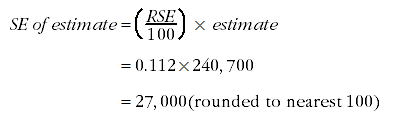7 Therefore, there are about two chances in three that the actual number of people who said the reason they did not take steps to limit their personal electricity use was because they did not care how much electricity was used was in the range of 213,700 to 267,700 and about 19 chances in 20 that the value was in the range of 186,700 and 294,700. This example is illustrated in the diagram below: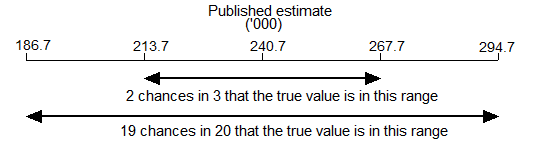PROPORTION AND PERCENTAGES

8 Proportions and percentages formed from the ratio of two estimates are also subject to sampling errors. The size of the error depends on the accuracy of both the numerator and the denominator. A formula to approximate the RSE of a proportion is given below. The formula is only valid when the numerator is a subset of the denominator: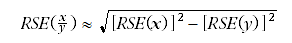9 As an example, using estimates from Table 6, of the 1,853,000 people who said they did not take steps to limit their personal electricity use, 13%, that is 240,700 people said it was because they did not care how much electricity was used. The RSE for 240,700 is 11.2% and the RSE for 1,853,000 is 3.0%. Applying the above formula, the approximate RSE of the proportion is: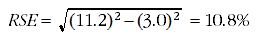10 Therefore, the SE for the proportion of people who said they did not take steps to limit their personal electricity use because they did not care how much electricity was used is 1.4 percentage points ((10.8/100) x 13). Hence, there are about two chances in three that the proportion of people who said they did not take steps to limit their personal electricity use because they did not care how much electricity was used is between 11.6% and 14.4%, and 19 chances in 20 that the proportion is between 10.2% and 15.8%.

DIFFERENCES

11 Published estimates may also be used to calculate the difference between two survey estimates (numbers or proportions). Such an estimate is also subject to sampling error. The sampling error of the difference between two estimates depends on their SEs and the relationship (correlation) between them. An approximate SE of the difference between two estimates (x-y) may be calculated by the following formula: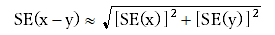12 While this formula will only be exact for differences between separate and uncorrelated characteristics or sub populations, it provides a good approximation for the differences likely to be of interest in this publication.

SIGNIFICANCE TESTING

13 A statistical significance test for any comparisons between estimates can be performed to determine whether it is likely that there is a difference between two corresponding population characteristics. The standard error of the difference between two corresponding estimates (x and y) can be calculated using the formula in paragraph 11. The standard error is then used to create the following test statistic: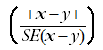14 If the value of this test statistic is greater than 1.96 then there is evidence, with a 95% level of confidence, of a statistically significant difference in the two populations with respect to that characteristic. Otherwise, it cannot be stated with confidence that there is a real difference between the populations with respect to that characteristic.

RELATIVE STANDARD ERROR TABLE

15 The RSEs for Table 6 are included below:

 Table 6 Reasons currently does not take steps to limit personal electricity use, Persons who do not currently take steps to limit electricity use, 2011–12 (a)Number ('000) Proportion (%) RSE of Number (%) RSE of Proportion (%) Electricity consumption already low enough 588.2 31.7 5.6 5.4 Haven't thought about saving electricity 281.3 15.2 10.5 9.9 Comfort 254.3 13.7 10.0 9.0 Inconvenient 252.4 13.6 8.9 7.9 Don't care how much electricity is used 240.7 13.0 11.2 10.7 Limiting electricity consumption not a priority 224.9 12.1 9.0 8.1 Lack of knowledge/don't know what to do 97.1 5.2 15.2 15.2 Time consuming 81.2 4.4 14.9 14.3 Not cost-effective 56.5 3.1 19.2 18.0 Already renewable *27.9 *1.5 29.9 30.4 Other 161.9 8.7 14.7 13.8Total(b) 1,853.0 .. 3.0 ..
* estimate has a relative standard error of 25% to 50% and should be used with caution
.. not applicable
(a) Excludes people not connected to an electricity supply.
(b) Components may not add to total as people may have reported more than one reason.